# Total Internal Reflection

## Total Internal Reflection

A critical concept in the case of optical fibers is the concept of total internal reflection. The bending of light as it goes from one medium into another is related to the index of reflection of the materials (n1 and n2). By Snell's law when n1 > n2 then θ 1 is less than θ2. As you decrease the angle θ1 and bring the light closer to parallel with the interface you reach a critical angle θ1c where there is total internal reflection.

Snells laws are also known as Fresnel's Equations: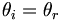$\theta _i = \theta _r\,\!$

and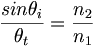$\frac {sin \theta_i} {\theta_t} = \frac {n_2} {n_1}\,\!$

where$\theta_i\,\!$ is the angle of incidence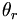$\theta_r\,\!$ is the angle of reflection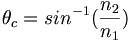$\theta_{c} = sin^{-1}(\frac {n_2} {n_1} )\,\!$

where: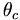$\theta_{c}\,\!$ is the critical angle$n_1 , n_2\,\!$ are the index of refraction of the two media

if θ1 keeps Increasing: θ2 approaches 90°

for a critical angle Θ1, Θ2 = 90°and the beam emerges along the surface

When$\theta_2 = 90° \rightarrow sin \theta_2 = 1\,\!$$\theta_{1c} = sin^{-1} \left (\frac {n_2} {n_1} \right )\,\!$

and when$\theta_1 > \theta_{1c}\,\!$ there is total reflection

In this case there is no transmitted light in the second medium (the irradiance of the reflected and incident beams is equal). Light interacts at the interface and there is a phase shift between the incident and the reflected beams which is dependent on the two materials.

This is a critical process in optical fibers and waveguides because it can carry light for long distances. For these values of index of refraction you get a critical angle of 78.6 which means the light must be very nearly parallel to the interface.

for n1 = 1.53 and n2 = 1.50 : θ1c = 78.6°

### Simulations

This flash simulation shows how total internal reflection and minimum radius of turns depends on the diameter of the optical fiber. The diameter changes how much of the light is below the critical angle where it is fully reflected.

This simulation allows you to select combinations of materials with different indexes of refraction. Try to get the best combination to achieve total internal reflection.